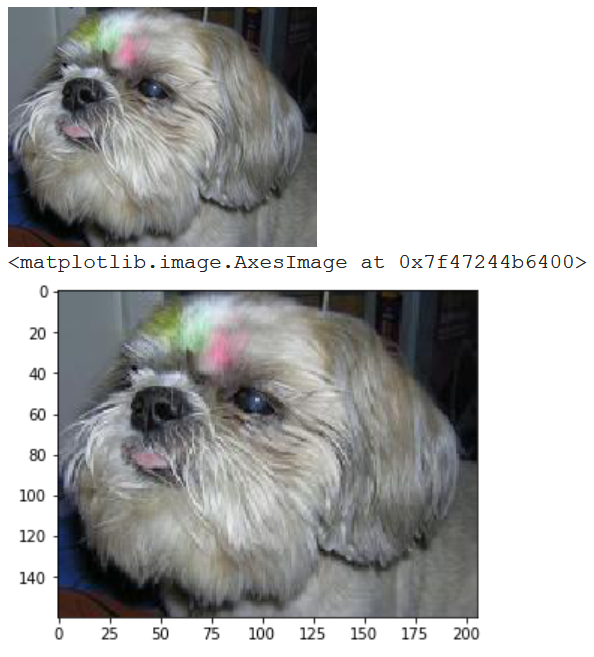Matplotlib is one of the default choices when plotting images in Python and should always be considered first.

There are two interesting libraries you can read images and transform them. Both these work from Jupyter notebooks. These are PIL and OpenCV.

(I) PIL library that also has handy display method.

%matplotlib inline
import PIL
from PIL import Image
import matplotlib.pyplot as plt
import torchvision

img = PIL.Image.open("/data/image1234.JPEG")
img.show()  # will open in external program
display(img) # display on any frontend

ToTensor = torchvision.transforms.ToTensor()
FromTensor = torchvision.transforms.ToPILImage()
t = FromTensor(ToTensor(img))
plt.imshow(t) # matplotlib


The program above will show the following:(II) OpenCV is another option to work with images in Python. (It also supports Videos)

%matplotlib inline
import cv2
import matplotlib.pyplot as plt

img = "/data/image1234.JPEG"